# 55 Work with Dates and Times

 <  Day Day Up  >

Calc supports almost every national and international date and time format. Calc converts date and time values that you type to a special internal number that represents the number of days since midnight January 1, 1900. Although this strange internal date representation of days since 1-1-1900 might not make sense at first, you'll use these values to compute time between two or more dates. You can easily determine how many days an account is past due, for example, by subtracting the current date from the cell in the worksheet that contains the due date.

Before You Begin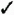41 Enter Simple Data into a Spreadsheet52 Enter Calc Functions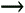59 Format Cells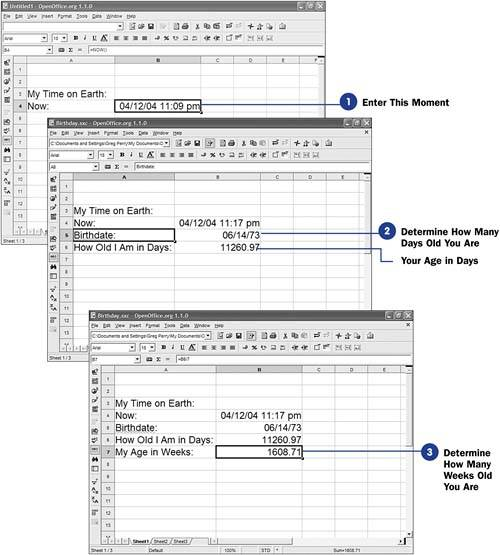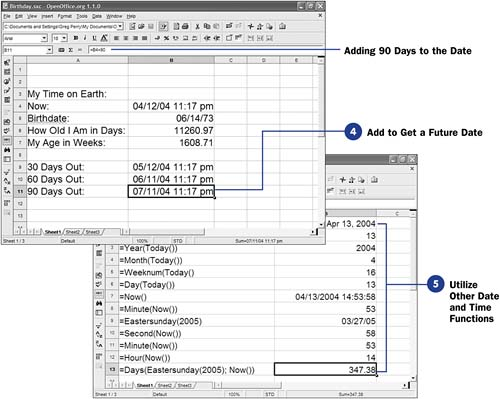If you enter a date in a longer format, such as July 4, 1776 , Calc usually converts the date to another format (such as 7/4/76 18:15 ). You can enter a date, a time value, or both. You can format the date and time values you enter (see 59 Format Cells ) to take on any format you wish.

TIP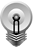The date and time functions are useful for calculating durations for past due and other calculations related to date and time values.

1. Enter This Moment

Type =Now() in a cell. When you press Enter , Calc converts the function to the computer's currently set date and time. You can use this to calculate values based on this moment, such as the number of days old you are.

TIPRemember that Now() and the other date functions operate as number of days since January 1, 1900. Therefore, when you add or subtract dates, with or without using Calc's date functions, the result is always a number of days.
2. Determine How Many Days Old You Are

Enter your birth date. Type the date in any format. Although you can format the date using the Format menu, don't worry about the format now; concentrate on what occurs when you use date arithmetic.

Subtract your birth date from the current date to determine how many days old you are. Digits to the right of the decimal indicate partial days since midnight of your birth date.

NOTE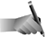Generally, you'll use date formats to hide the time when you work with date arithmetic. When working with time values, a day has 24 hours in it, so you'll need to multiply by 24 to obtain the number of hours represented by the value. When you subtract two date and time values, if you multiply by 24 you'll get the number of hours represented. For example, if you subtract a cell containing your birth date from a cell containing today's date, then multiply the result by 24, you'll learn how old you are in hours.
3. Determine How Many Weeks Old You Are

Because the dates work in days, you can divide your age in days by seven to determine approximately how old you are in weeks.

4. Add to Get a Future Date

What will be the date one month from today? Sure, it's simple to look at a calendar, but when you write general-purpose spreadsheets, you've got to be able to apply such formulas to dates to age accounts receivables and other calculations.

By adding 30, 60 , and 90 to today's date, you can display the date when future payments will come due. Calc understands how many days different months have in them, so adding 60 to April 12th properly returns June 11th and not June 12th.

5. Utilize Other Date and Time Functions

Several date and time functions are available to make working with dates and times simpler. Today() returns the date only (without the time, unlike Now() ). Day() returns today's day of the month of whatever date you use as its argument. Weeknum() returns the week number (within the year, from 1 to 52) of the date inside its argument list. Weeknum() requires two arguments: a date and either 1 or 2 to indicate that the start of the week is Sunday or Monday , respectively. Month() returns the month number of its date argument. Year() returns the year of the date given as its argument. You'll use these functions to pick off what you want to work with in another formula or label: either the day, month, or year by itself instead of working with the complete date.

Eastersunday() returns the date of Easter given the year of its argument. You can add to or subtract from Eastersunday() to get the days around the holiday, such as Good Friday, which displays for any year with the following calculation: =Eastersunday(yearCell) - 2.

TIPYou can enter a time value, a date value, or both. If you don't enter a date with a time value, Calc displays only the time in the cell.
Second() returns the second number of its time argument. Minute() returns the minute number of its time argument, and Hour () returns the hour number of its time argument.

Days() requires two date arguments and returns the number of days (and partial days) between two dates. Therefore, to determine the number of days between today and Easter, you could enter the following:

` `

`  =Days(Eastersunday(Year(Today())+1); Now())  `

Although at first glance this appears convoluted, it's a simple set of arguments.

 <  Day Day Up  >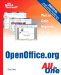Sams Teach Yourself OpenOffice.org All In One
ISBN: 0672326183
EAN: 2147483647
Year: 2003
Pages: 205
Authors: Greg Perry

Similar book on Amazon